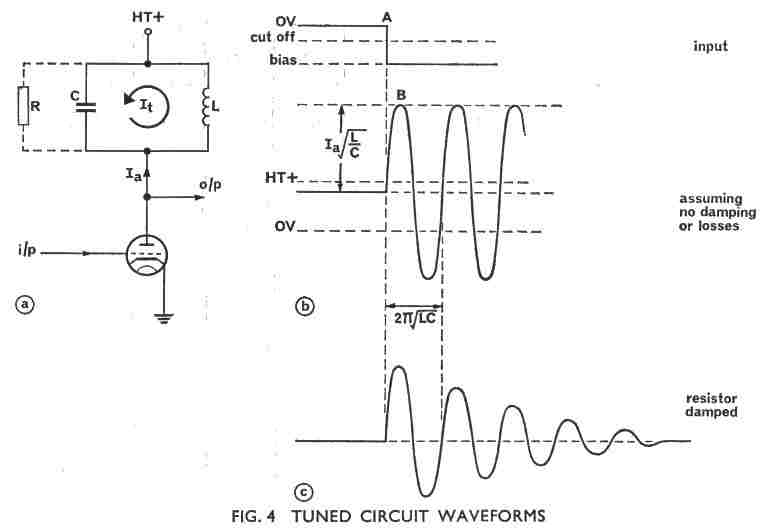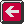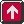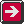Main Radar Home Radar theory Home AP3302 Pt3 Contents AP3302 Pt3 Section 2Contents AP 3302 Pt. 3 Section 2 CHAPTER 3 Square Waves applied to LR and LC circuitsAssuming that the valve has been switched on for some time, the whole of the anode current Ia will be flowing through the inductance L. The anode voltage will be at approximately HT, as the only losses are due to the small built-in resistance of the inductor. At instant A the valve is suddenly cut off and the supply of current ceases. However, the current flowing in an inductance cannot change instantaneously so the current Ia will continue to flow in the tuned circuit following the path shown as It in Fig 4a. This causes the capacitor C to charge up, and the voltage on C will begin to oppose the flow of current It. After a time depending on the tuned circuit constants, current flow ceases and the capacitor is fully charged. (Point B in Fig 4b). The voltage on the capacitor now causes the current It to build up in the opposite direction through the inductor, until maximum current Ia is again flowing and the voltage on the capacitor is again zero. The oscillatory action will then carry on at the tuned circuit resonant frequency as there are assumed to be no losses. What this really means is that at the instant of switch off we had a given amount of energy stored in the inductor (1/2LI2): this energy was then transferred to the capacitor (1/2CV2), then back to the inductor and so on. The rate of transfer is such that the oscillating output voltage is at the frequency of the tuned circuit 1/2p(sq rt LC). The output voltage depends on the value of the initial current flowing in the circuit, but it also depends on the L/C ratio and quite high voltages can be obtained from a circuit arrangement of this type. Two applications are worth mentioning, the ignition system of a motor car and a circuit called the ringing oscillator which is often used to produce high voltage trigger pulses in radar. Figure 4c shows the effect on the output of having a resistance in parallel with the tuned circuit and thus represents the practical case where the circuit is loaded by the following stage. As can be seen the amplitude of the output waveform decreases and the circuit is said to be damped. The lower the value of resistance in parallel with the tuned circuit, the faster the oscillations die away.Previous page To top of this page Next Page

Constructed by Dick Barrett
Email: